# 使用 COMSOL Multiphysics® 模拟 COVID-19 的传播

2020年 4月 7日

### COVID-19 的数学模型

S =易感者

E =潜伏者

I =感染者

R =康复并具有免疫力的人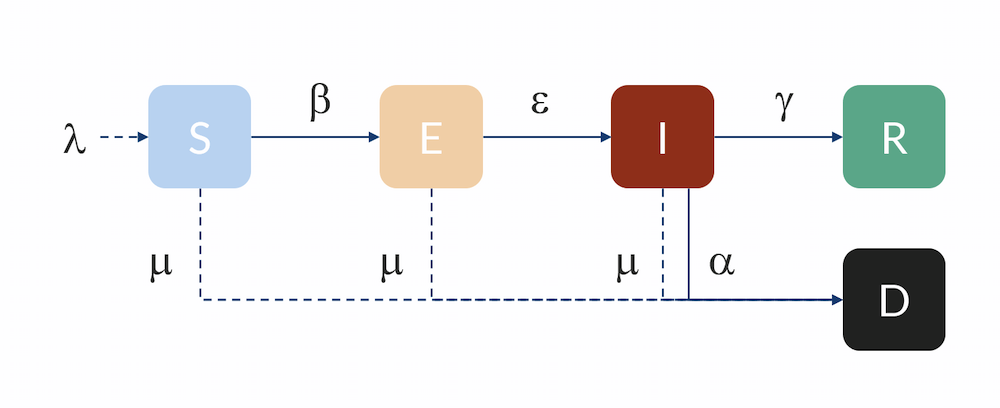(1)

${r_{nE}} = \frac{\beta }{N}SI$

β（单位：1人 /天）与基本传染数0和感染者具有传染性的平均天数（在被隔离或自我隔离之前）有关，nid

(2)

$\beta = \frac{{{R_0}}}{{{n_{id}}}}$

0  称为基本传染数（无量纲），它描述了感染者在康复之前与易感人的每次接触（当人群中完全没有免疫力时）的疾病传播。任何缓解或隔离措施都旨在通过减少传染率 β 或及时隔离感染者，来降低传染数。

(3)

$\frac{{dS}}{{dt}} = – \frac{\beta }{N}SI$

(4)

$\frac{{dE}}{{dt}} = \frac{\beta }{N}SI – \varepsilon E$

(5)

$\gamma = \frac{1}{{{n_{id}}}}$

(6)

$\frac{{dI}}{{dt}} = \varepsilon E – \gamma I – \alpha I$

(7)

$\frac{{dR}}{{dt}} = \gamma I$

(8)

$\frac{{dD}}{{dt}} = \alpha I$

### 展平曲线

N = 100 万个人

0 = 2.25，基本传染数

Ñ ID =5 天

0  = N –  0，易感人群

0  = 10 个感染者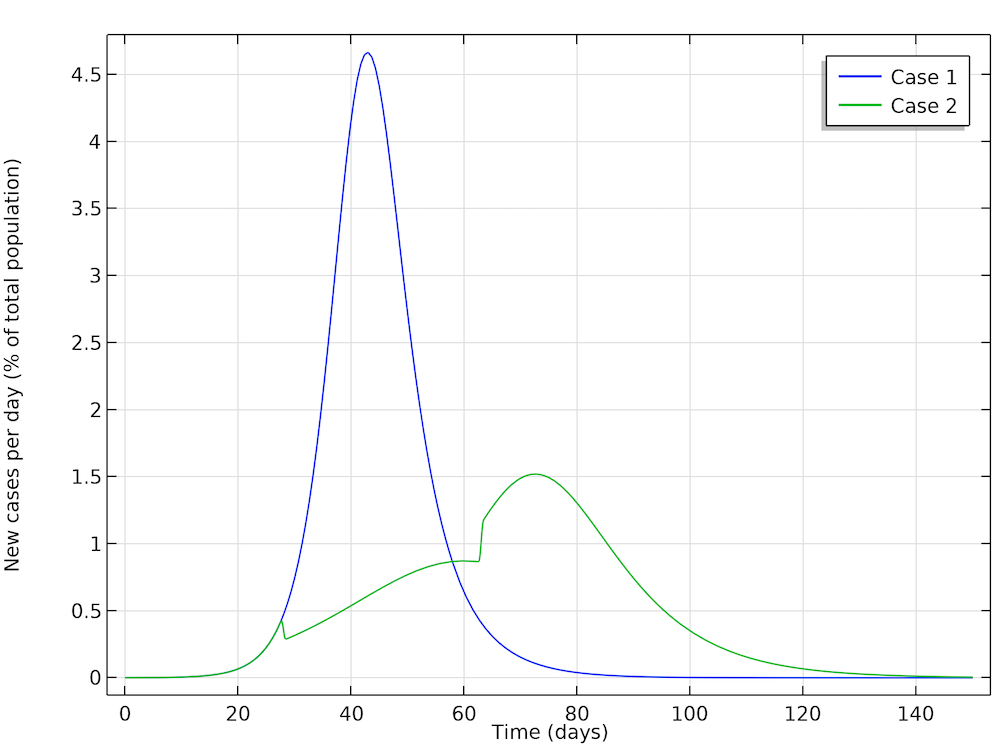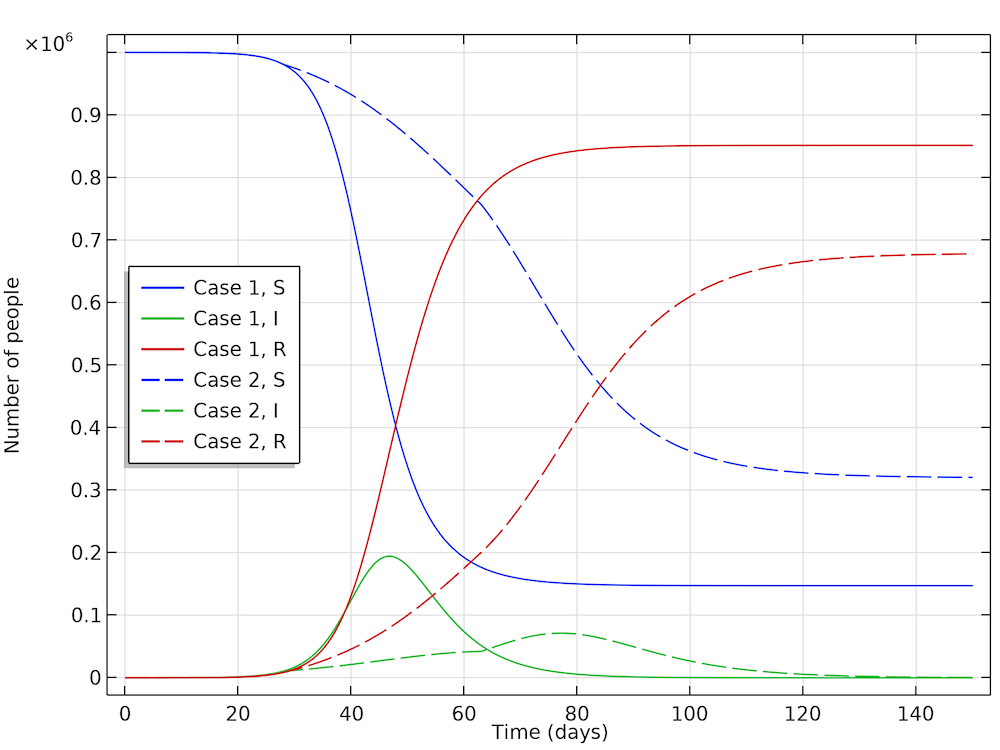### 考虑不同人群分布的流行病发展过程计算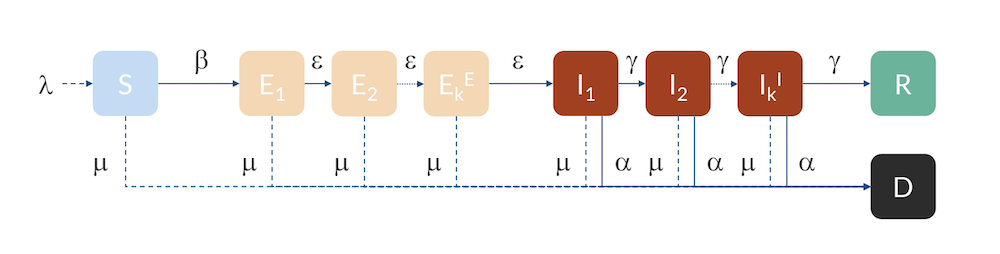### COVID-19在中国湖北的传播模拟

2019年1月22日，当局实施了封锁措施，并在该月底进行了测量，结果显示基本传染数明显下降（参考文献5） 。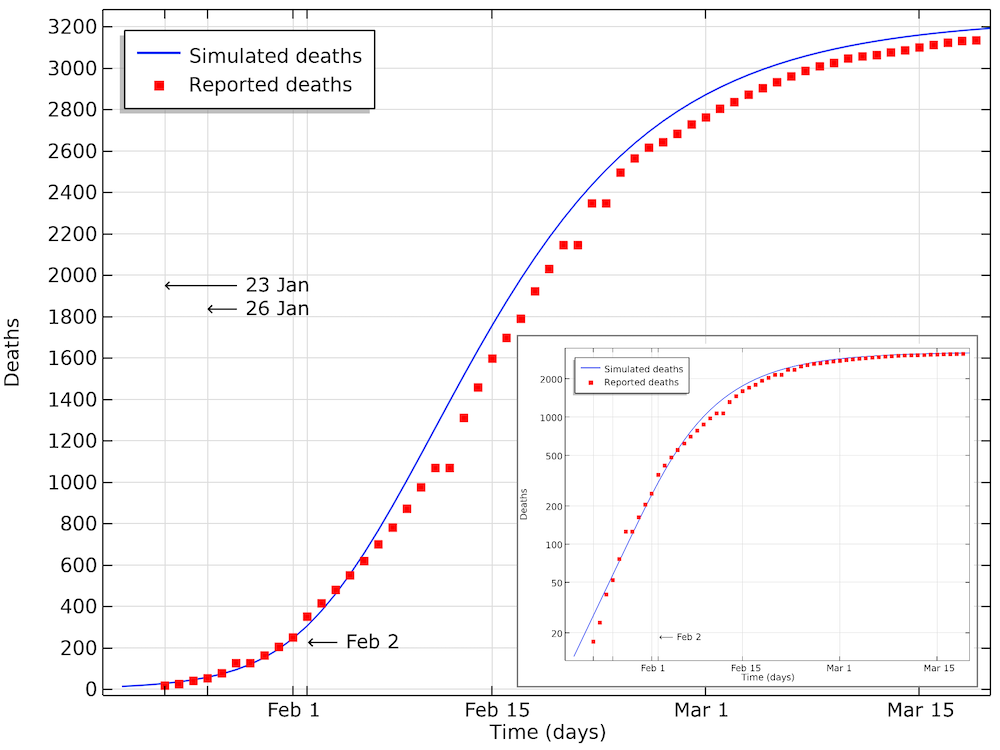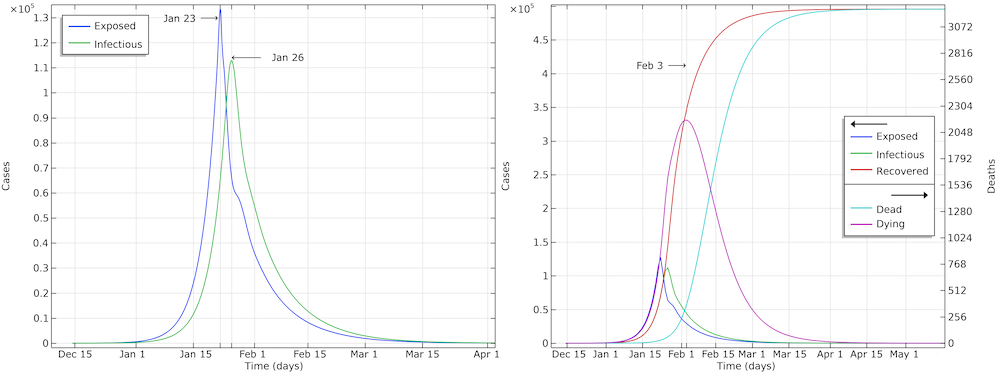### 瑞典：保持社交距离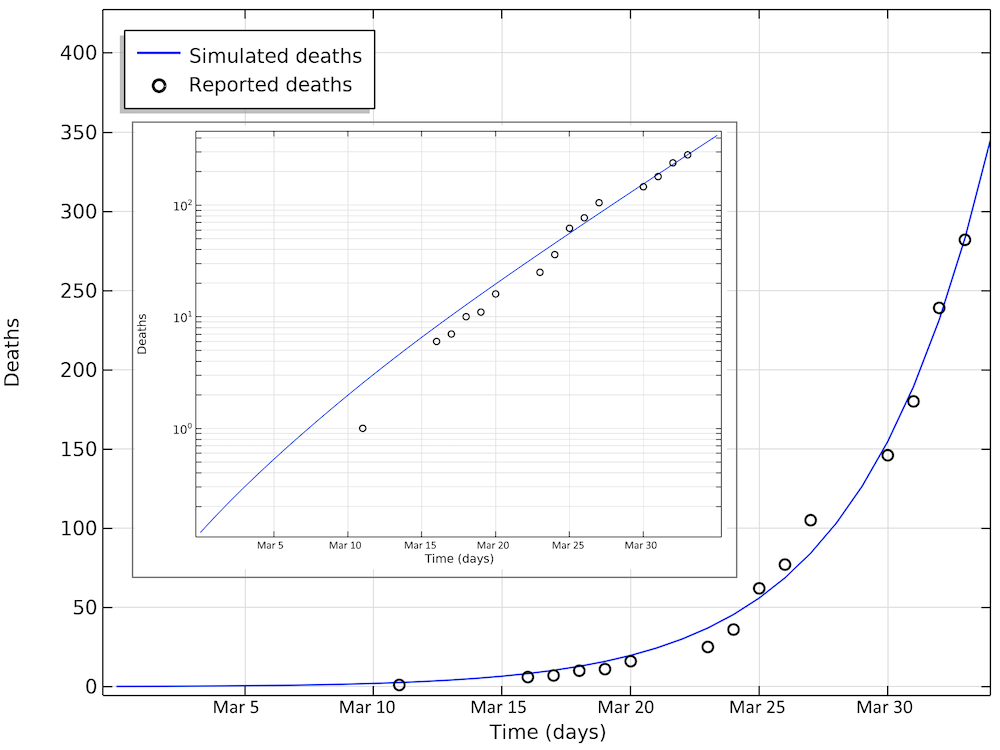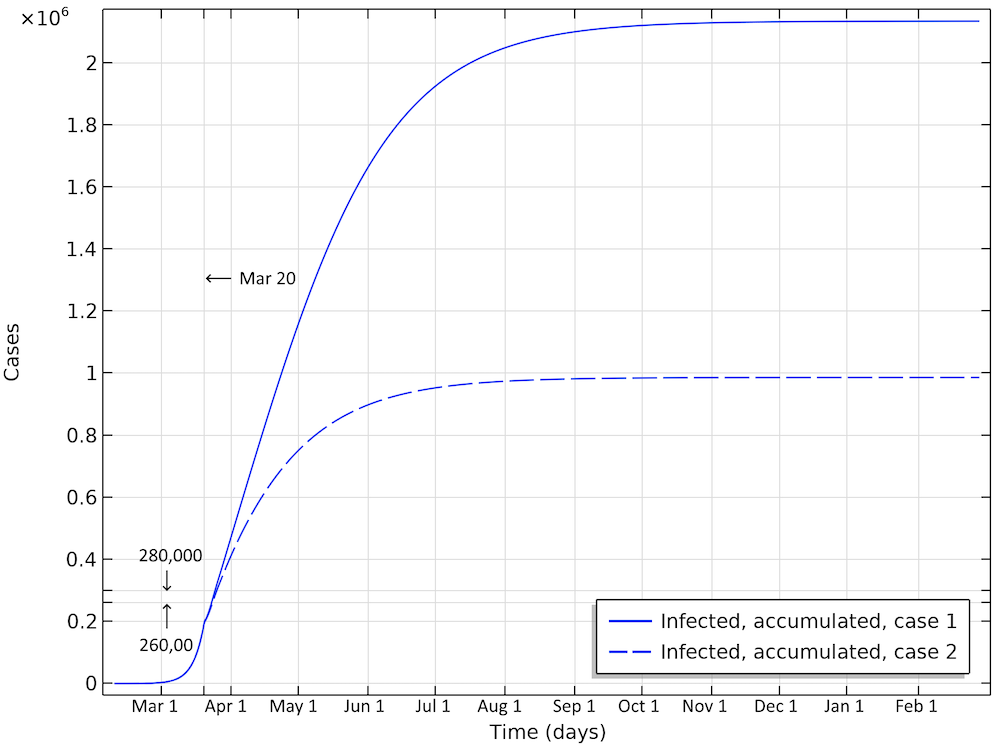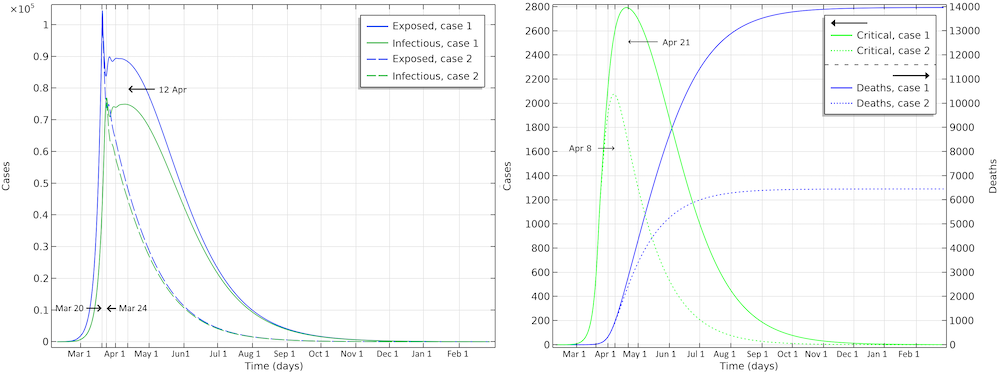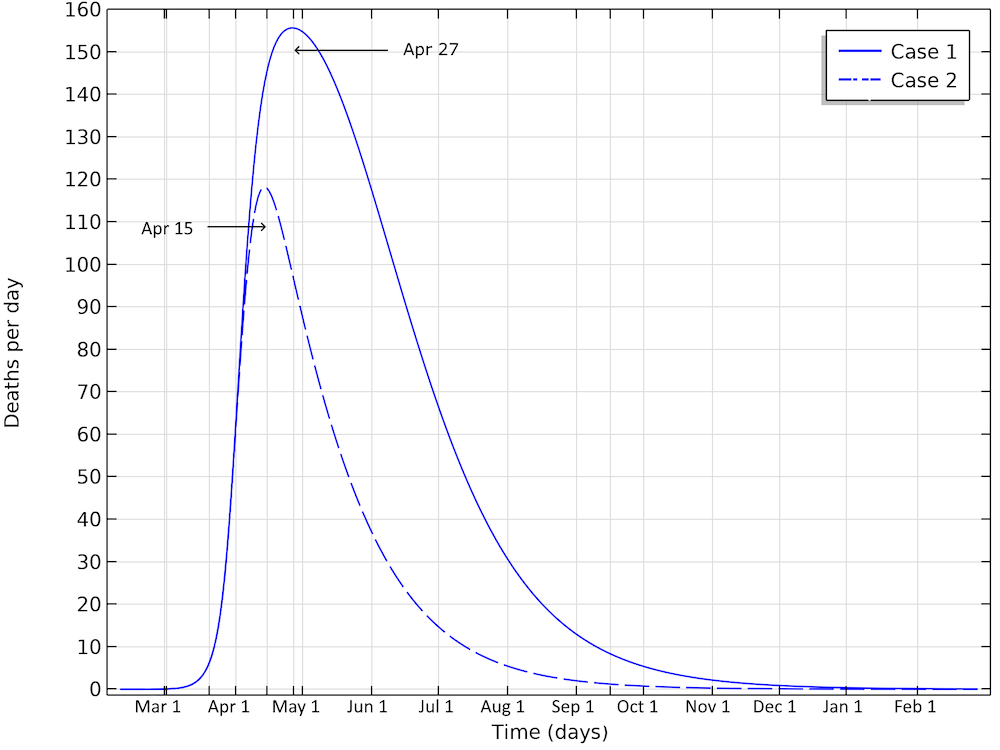### 美国：大规模隔离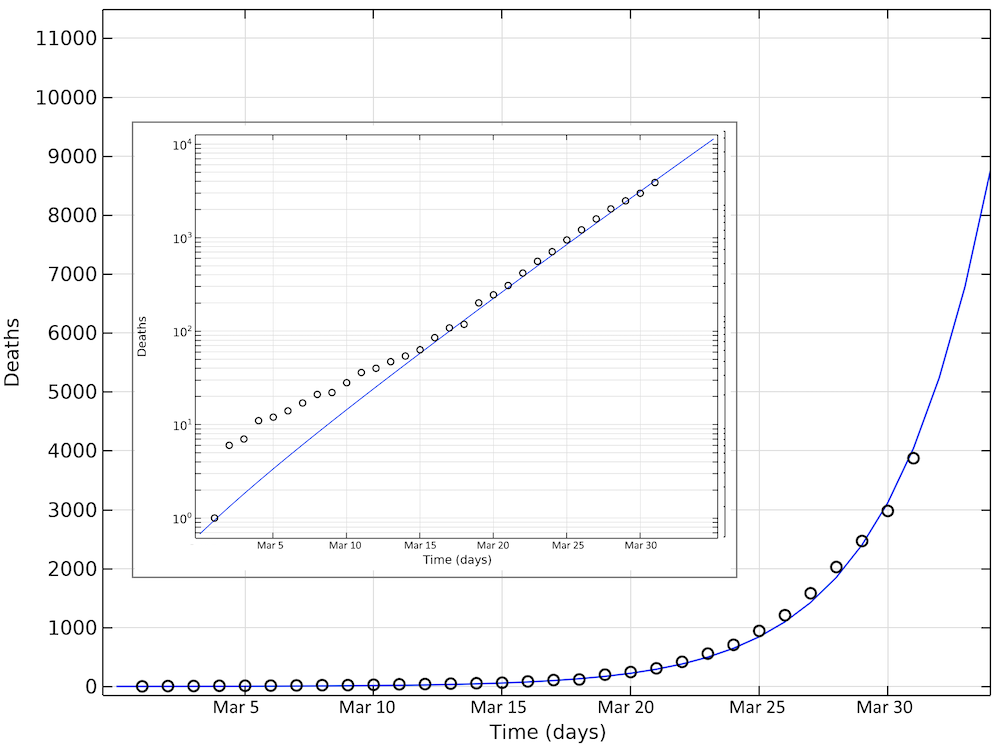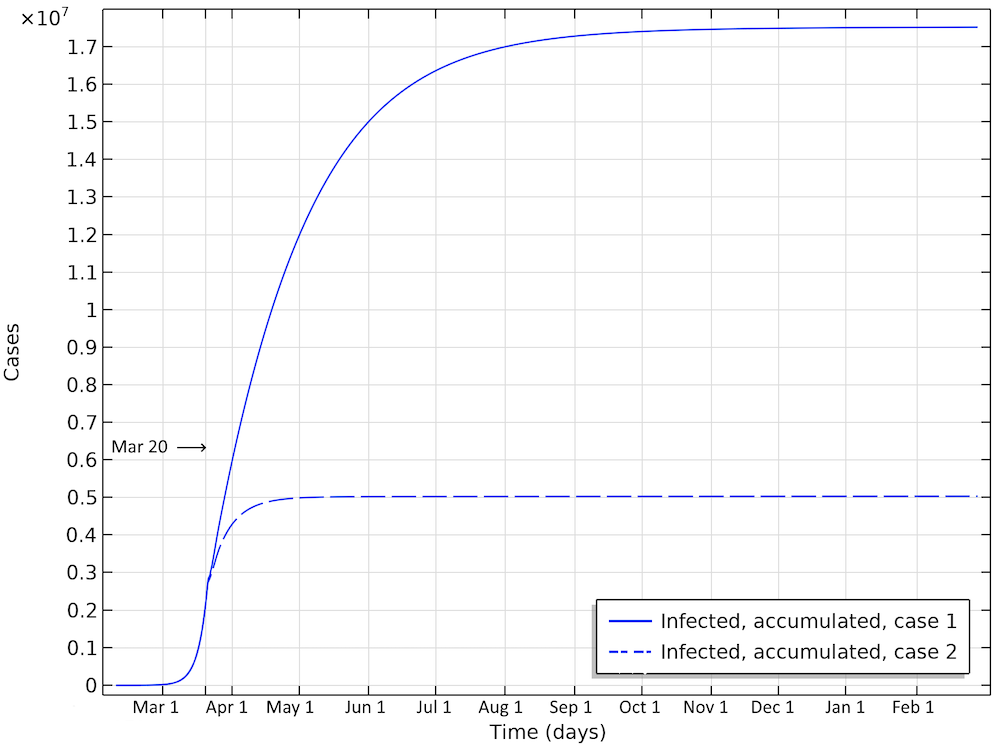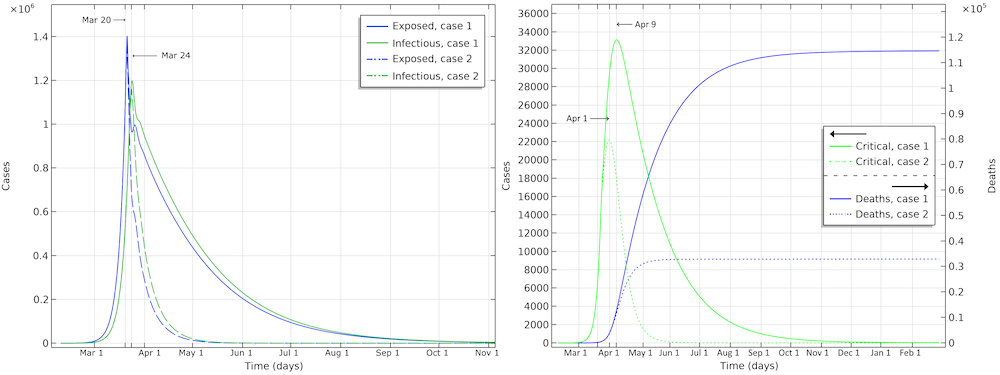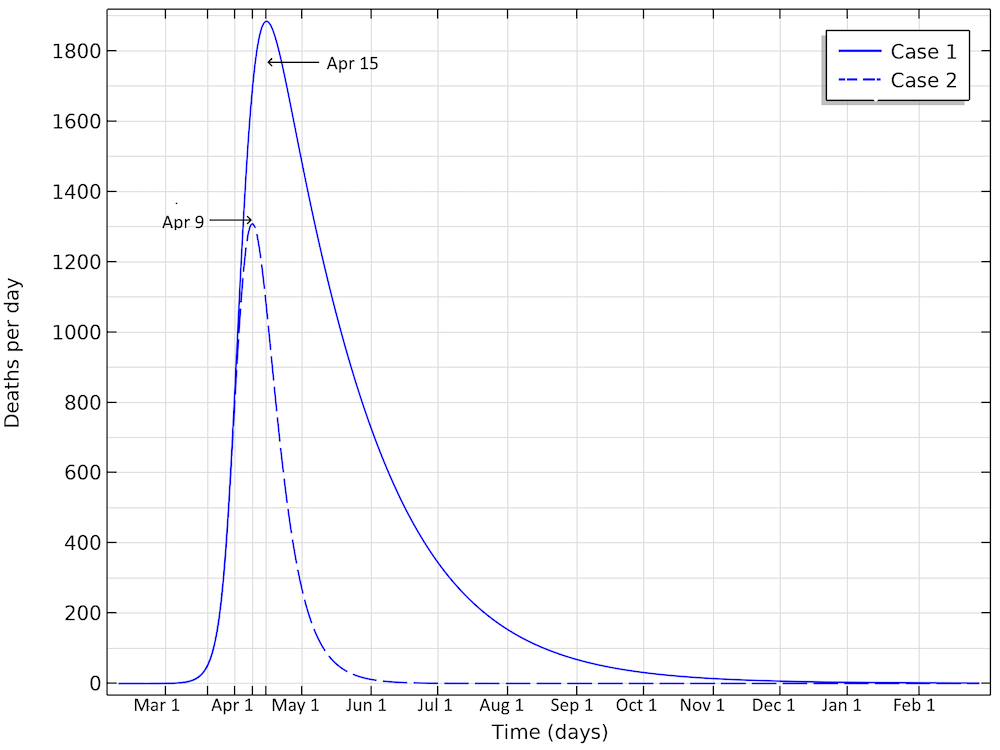### 下载模型和应用文件

1. H. Weiss, “The SIR model and the Foundations of Public Health”, MATerials MATemàtics, vol. 2013, no. 3, pp. 1–17, 2013.
2. M. Höhle, “Flatten the COVID-19 curve”, Theory meets practice…, 2020.
3. W.M. Getz and E.R. Dougherty, “Discrete Stochastic Analogs of Erlang Epidemic Models”, Journal of Biological Dynamics, vol. 12, pp.16–38, 2018.
4. R. Verity, L.C. Okell, I. Dorigatti, P. Winskill, C. Whittaker, et al., “Estimates of the severity of coronavirus disease 2019: a model-based analysis”, The Lancet Infectious Diseases, 2020.
5. A.J. Kucharski, T.W. Russell, C. Diamond, Y. Liu, J. Edmunds, S. Funk, R.M. Eggo, “Early dynamics of transmission and control of COVID-19: a mathematical modelling study”, The Lancet Infectious Diseases, 2020.
6. R. Li, S. Pei, B. Chen, et at., “Substantial undocumented infection facilitates the rapid dissemination of novel coronavirus (SARS-CoV2)”, Science, 2020.
7. Y. Liu, A.A. Gayle, A. Wilder-Smith, and J. Rocklöv, “The reproductive number of COVID-19 is higher compared to SARS coronavirus”, Journal of Travel Medicine, vol. 27, no. 2, 2020.